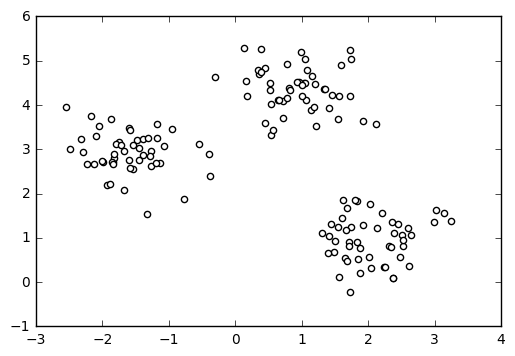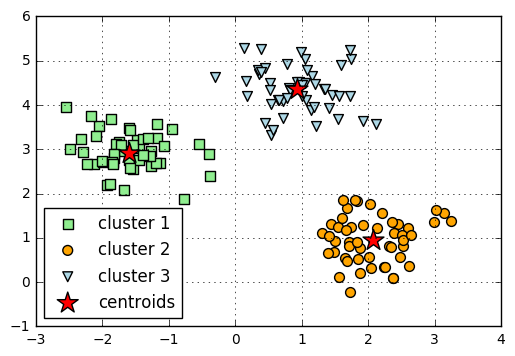# Kmeans: k-means clustering

A implementation of k-means clustering.

from mlxtend.cluster import Kmeans

## Overview

Clustering falls into the category of unsupervised learning, a subfield of machine learning where the ground truth labels are not available to us in real-world applications. In clustering, our goal is to group samples by similarity (in k-means: Euclidean distance).

The k-means algorithms can be summarized as follows:

1. Randomly pick k centroids from the sample points as initial cluster centers.
2. Assign each sample to the nearest centroid $\mu(j), \; j \in {1,...,k}$.
3. Move the centroids to the center of the samples that were assigned to it.
4. Repeat steps 2 and 3 until the cluster assignments do not change or a user-defined tolerance or a maximum number of iterations is reached.

## Example 1 - Three Blobs

import matplotlib.pyplot as plt
from mlxtend.data import three_blobs_data

X, y = three_blobs_data()
plt.scatter(X[:, 0], X[:, 1], c='white')
plt.show()#### Compute the cluster centroids:

from mlxtend.cluster import Kmeans

km = Kmeans(k=3,
max_iter=50,
random_seed=1,
print_progress=3)

km.fit(X)

print('Iterations until convergence:', km.iterations_)
print('Final centroids:\n', km.centroids_)

Iteration: 2/50 | Elapsed: 00:00:00 | ETA: 00:00:00

Iterations until convergence: 2
Final centroids:
[[-1.5947298   2.92236966]
[ 2.06521743  0.96137409]
[ 0.9329651   4.35420713]]


#### Visualize the cluster memberships:

y_clust = km.predict(X)

plt.scatter(X[y_clust == 0, 0],
X[y_clust == 0, 1],
s=50,
c='lightgreen',
marker='s',
label='cluster 1')

plt.scatter(X[y_clust == 1,0],
X[y_clust == 1,1],
s=50,
c='orange',
marker='o',
label='cluster 2')

plt.scatter(X[y_clust == 2,0],
X[y_clust == 2,1],
s=50,
c='lightblue',
marker='v',
label='cluster 3')

plt.scatter(km.centroids_[:,0],
km.centroids_[:,1],
s=250,
marker='*',
c='red',
label='centroids')

plt.legend(loc='lower left',
scatterpoints=1)
plt.grid()
plt.show()## API

Kmeans(k, max_iter=10, convergence_tolerance=1e-05, random_seed=None, print_progress=0)

K-means clustering class.

Parameters

• k : int

Number of clusters

• max_iter : int (default: 10)

Number of iterations during cluster assignment. Cluster re-assignment stops automatically when the algorithm converged.

• convergence_tolerance : float (default: 1e-05)

Compares current centroids with centroids of the previous iteration using the given tolerance (a small positive float)to determine if the algorithm converged early.

• random_seed : int (default: None)

Set random state for the initial centroid assignment.

• print_progress : int (default: 0)

Prints progress in fitting to stderr. 0: No output 1: Iterations elapsed 2: 1 plus time elapsed 3: 2 plus estimated time until completion

Attributes

• centroids_ : 2d-array, shape={k, n_features}

Feature values of the k cluster centroids.

• custers_ : dictionary

The cluster assignments stored as a Python dictionary; the dictionary keys denote the cluster indeces and the items are Python lists of the sample indices that were assigned to each cluster.

• iterations_ : int

Number of iterations until convergence.

Examples

For usage examples, please see https://rasbt.github.io/mlxtend/user_guide/classifier/Kmeans/

### Methods

fit(X, init_params=True)

Learn model from training data.

Parameters

• X : {array-like, sparse matrix}, shape = [n_samples, n_features]

Training vectors, where n_samples is the number of samples and n_features is the number of features.

• init_params : bool (default: True)

Re-initializes model parameters prior to fitting. Set False to continue training with weights from a previous model fitting.

Returns

• self : object

predict(X)

Predict targets from X.

Parameters

• X : {array-like, sparse matrix}, shape = [n_samples, n_features]

Training vectors, where n_samples is the number of samples and n_features is the number of features.

Returns

• target_values : array-like, shape = [n_samples]

Predicted target values.